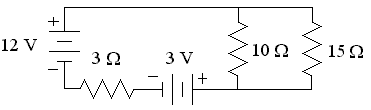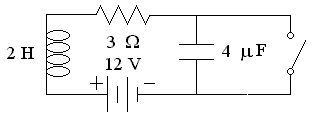First Name: ___________________ Last Name: ____________________ Section: _________

May 14, 1999 Physics 208

Final Exam

Print your name and section clearly on all nine pages. (If you do not know your section number, write your TAs name.) Show all work in the space immediately below each problem. Your final answer must be placed in the box provided. Problems will be graded on reasoning and intermediate steps as well as on the final answer. Be sure to include units wherever necessary, and the direction of vectors. Each problem is worth 25 points. In doing the problems, try to be neat. Check your answers to see that they have the correct dimensions (units) and are the right order of magnitudes. You are allowed two 8½ x 11" sheet of notes and no other references. The exam lasts exactly 2 hours.

(Do not write below)

SCORE:

Problem 1: __________

Problem 2: __________

Problem 3: __________

Problem 4: __________

Problem 5: __________

Problem 6: __________

Problem 7: __________

Problem 8: __________

TOTAL: ___________

First Name: ___________________ Last Name: ____________________ Section: _________
1. An insulated ball with a radius of 10 cm has an electric charge of 2 nC distributed uniformly throughout its interior.
a. What is the electric field 8 cm from the center of the ball? (9 pts.)

b. What is the electric potential (relative to infinity) 12 cm from the center of the ball? (8 pts.)

c. What is the force on an electron 15 cm from the center of the ball? (8 pts.)

First Name: ___________________ Last Name: ____________________ Section: _________
1. Consider the circuit below:

2.a. What is the voltage across the 3-ohm resistor? (10 pts.)

b. What is the current in the 15-ohm resistor? (15 pts.)

First Name: ___________________ Last Name: ____________________ Section: _________

3. A long, straight wire carries a current of 100 A.

4. a. What is the magnitude of the magnetic field 5 cm from the wire? (8 pts.)

b. What is the force on an electron 5 cm from the wire moving in the direction of the current with kinetic energy of 100 eV? (8 pts.)

c. If the current is turned uniformly to zero in a time of 500 ?S, what voltage is induced in a single-turn circular loop with an area of 1 cm2, 5 cm from the wire, and axis parallel to the magnetic field? (9 pts.)

First Name: ___________________ Last Name: ____________________ Section: _________

5. In the circuit below, the switch has been closed for a long time and is then opened at t = 0.
6.a. What is the current in the resistor just before the switch is opened? (5 pts.)

b. At what frequency (in Hz) does the circuit oscillate after the switch is opened? (5 pts.)

c. What is the maximum instantaneous power dissipated by the resistor? (5 pts.)

d. What is the maximum voltage across the inductor? (5 pts.)

e. What is the voltage across the capacitor after the switch has been open for a long time? (5 pts.)

First Name: ___________________ Last Name: ____________________ Section: _________

7. Assume a 100-W light bulb radiates light with an average wavelength of 600 nm isotropically in all directions.

8. a. What is the rms electric field 50 cm from the bulb? (5 pts.)

b. What is the rms magnetic field 50 cm from the bulb? (5 pts.)

c. What is the radiation pressure on a black surface 50 cm from the bulb? (5 pts.)

d. What is the energy (in eV) of the photons emitted by the bulb? (5 pts.)

e. How many photons are emitted by the bulb each second? (5 pts.)

First Name: ___________________ Last Name: ____________________ Section: _________

9. Astronauts leave two lasers (? = 650 nm) 1 km apart on the moon pointed toward the earth. The earth-moon distance is 3.84 x 108 m.

10. a. What diameter telescope is required to just resolve the laser beams from the earth? (9 pts.)

b. If the telescope lens has a focal length of 140 cm, how far apart are the images of the lasers? (8 pts.)

c. If these images are magnified by a second converging lens with a focal length of 2 cm, how far from the second lens must a photographic plate be placed so that the images of the lasers are 1 mm apart? (8 pts.)

First Name: ___________________ Last Name: ____________________ Section: _________

11. Consider an electron in the 1s state of a neutral Uranium atom (Z = 92) in its ground state.

12. a. What is the kinetic energy of this electron in eV? (6 pts.)

b. What is the relativistic ?-factor of this electron? (6 pts.)

c. What is the de Broglie wavelength of the electron? (7 pts.)

d. What wavelength photon is required to eject this electron from the atom? (6 pts.)

First Name: ___________________ Last Name: ____________________ Section: _________

13. A smoke detector uses Americium-243 (Z = 95) which decays to Neptunium by ?-decay with a half life of 7370 years. The Neptunium quickly ?--decays to Plutonium whose half life is much greater than Americium.
a. What is the charge (Z) and atomic mass (A) of the Neptunium nucleus? (5 pts.)

b. What is the charge (Z) and atomic mass (A) of the Plutonium nucleus? (5 pts.)

c. What fraction of the Americium decays in 100 years? (5 pts.)

d. If the mass of Americium is 1 g, what is its radioactivity (in Curies)? (5 pts.)

e. What other lepton is produced in the above decay process? (5 pts.)